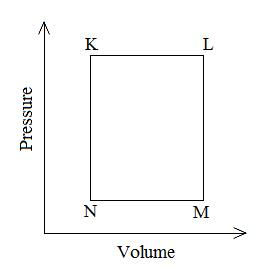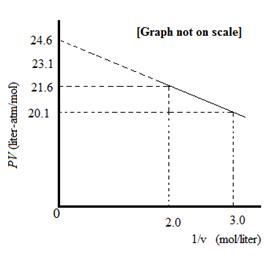Courses

# Notes | EduRev

## JEE : Notes | EduRev

The document Notes | EduRev is a part of the JEE Course NEET Revision Notes.
All you need of JEE at this link: JEE

Question 1: A fixed mass ‘m’ of a gas is subjected to transformation of states from K to L to M to N and back to K as shown in the figureThe pair of isochoric processes among the transformation of states is    (IIT JEE-2013)
(a) K to L and L to M
(b) L to M and N to K
(c) L to M and M to N
(d) M to N and N to K
Answer: b
Solution: Plot for the processes N to K and L to M are a straight line. This indicates that the value of V remains same at all the points during these processes. These processes take place at constant volume, so these are isochoric processes.
Hence, the correct option is b.

Question 2: For one mole of a van der Waals gas when b = 0 and T= 300 K, the PVvs. 1/V plot is shown below. The value of the van der Waals constant ‘a’ (atm.litre2 mol–2) is    (IIT JEE 2012)(a) 1.0
(b) 4.5
(c) 1.5
(d) 3.0
Answer: c
Solution:
Van der waals equation for 1 mole of real gas:
(P+a/v2)(v-b) =RT
Given that, b=0
So, the equation becomes;
(P+a/v2)v =RT
or
pv + a/v = RT
or
pv = -a×1/v +RT
Comparing with equation of straight line: y = mx +c, it can be concluded that
pv vs 1/v graph would be a straight line with a negative slope equal to a.
Thus, slope of the plot = a = (y2-y1)/(x2-x1) = (21.6-20.1)/(3-2) =1.5
Hence, the correct option is c.

Question 3: To an evacuated vessel with movable piston under external pressure of 1 atm, 0.1 mol of He and 1.0 mol of an unknown compound (vapour pressure 0.68 atm.at 0oC) are introduced. Considering the ideal gas behaviour, the total volume (in litre) of the gases at 0oC is close to    (IIT-JEE 2011)
Solution:
The unknown compound would not follow the ideal gas equation and thus the problem can
be solved by applying the ideal gas equation to the He gas only.
Using ideal gas equation:
V = (nRT )/P
V = (0.1 × 0.0821 × 273)/(0.32)
V = 7 L

Offer running on EduRev: Apply code STAYHOME200 to get INR 200 off on our premium plan EduRev Infinity!

291 docs

,

,

,

,

,

,

,

,

,

,

,

,

,

,

,

,

,

,

,

,

,

;